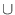Next: Dynamic Integer Sets ( Up: Basic Data Types Previous: Sets ( set ) ~ Contents ~ Index

# Integer Sets ( int_set )

Definition

An instance S of the data type int_set is a subset of a fixed interval [a..b] of the integers, called the range of S.

#include < LEDA/core/int_set.h >

Creation

 int_set S(int a, int b) creates an instance S of type int_set for elements from [a..b] and initializes it to the empty set. int_set S(int n) creates an instance S of type int_set for elements from [0..n - 1] and initializes it to the empty set.

Operations

 void S.insert(int x) adds x to S. Precondition a < = x < = b. void S.del(int x) deletes x from S. Precondition a < = x < = b. bool S.member(int x) returns true if x in S, false otherwise. Precondition a < = x < = b. int S.min() returns the minimal integer in the range of of S. int S.max() returns the maximal integer in the range of of S. void S.clear() makes S the empty set.

In any binary operation below, S and T must have the same range:

 int_set& S.join(const int_set& T) replaces S by ST and returns it. int_set& S.intersect(const int_set& T) ~ ~ replaces S by ST and returns it. int_set& S.diff(const int_set& T) replaces S by ST and returns it. int_set& S.symdiff(const int_set& T) ~ ~ replaces S by (ST)(TS) and returns it. int_set& S.complement() replaces S by [a..b]S and returns it. int_set S | const int_set& T returns the union of S and T. int_set S & const int_set& T returns the intersection of S and T. int_set S - const int_set& T returns the set difference of S and T. int_set S ~ int_set ~S returns the complement of S, i.e. [a..b]S.

Implementation

Integer sets are implemented by bit vectors. Operations insert, delete, member, min and max take constant time. All other operations take time O(b - a + 1).Next: Dynamic Integer Sets ( Up: Basic Data Types Previous: Sets ( set ) ~ Contents ~ Index